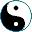<<
>>
This is probably the most difficult part of this presentation... Suppose we have two functors, F,G: XY. A natural transformation φ: F → G is defined when for each object
x ∈ X there is an arrow φ(x): F(x) → G(x) in Y, and we have the following property:

• for all f: a → b the equality is true: G(f) ∘ φ(a) = φ(b) ∘ F(f).

 F(a) F(f) F(b) → φ(a)↓ ↓φ(b) G(a) → G(b) G(f)

That's why it is called 'natural' - it acts consistently with the functors actions on arrows.